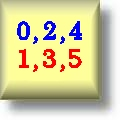Logic Worksheets

Dynamic Logic Worksheets for Teachers

Here is a graphic preview for all of the Logic worksheets. You can select different variables to customize these Logic worksheets for your needs. The Logic worksheets are randomly created and will never repeat so you have an endless supply of quality Logic worksheets to use in the classroom or at home. Our Logic worksheets are free to download, easy to use, and very flexible.

These Logic worksheets are a great resource for students in the 8th Grade through the 12th Grade.

Quick Link for All Logic Worksheets

Click the image to be taken to that Logic Worksheet.

Logic Definitions HandoutLogic Worksheets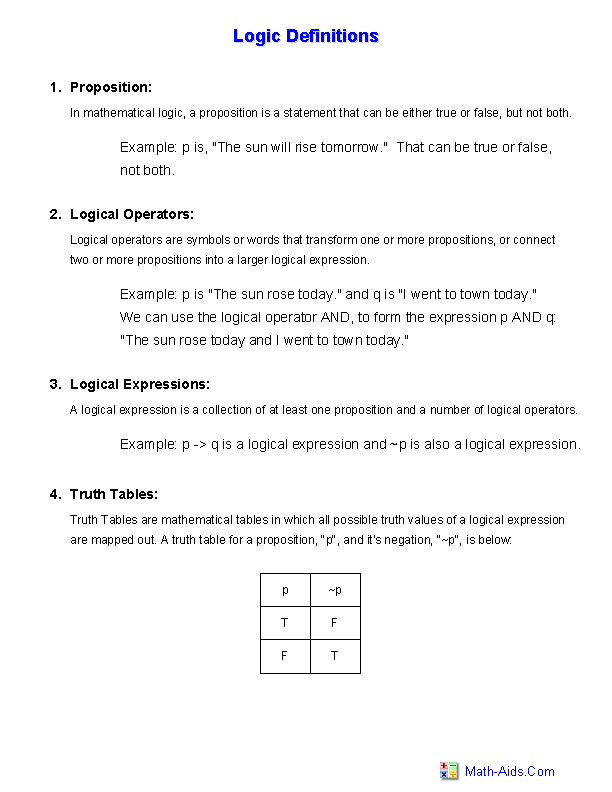Truth Table HandoutLogic Worksheets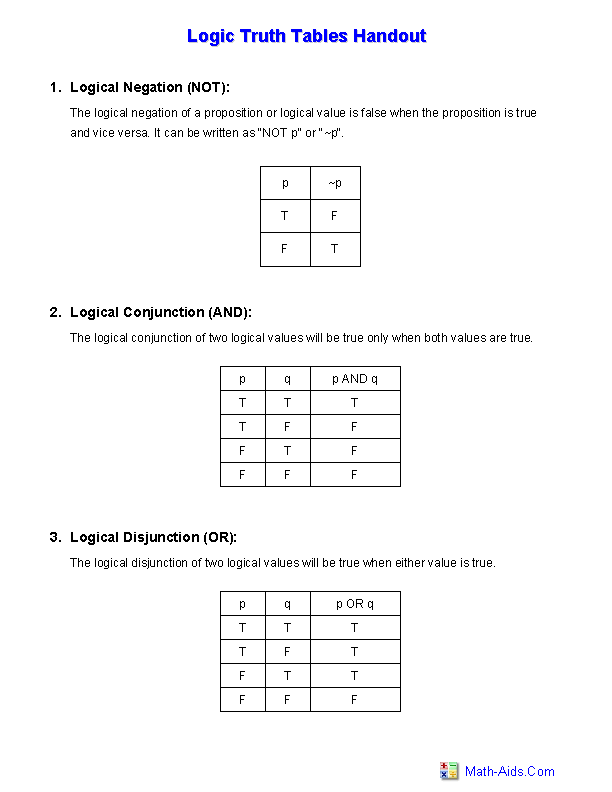Digital Truth Table HandoutLogic Worksheets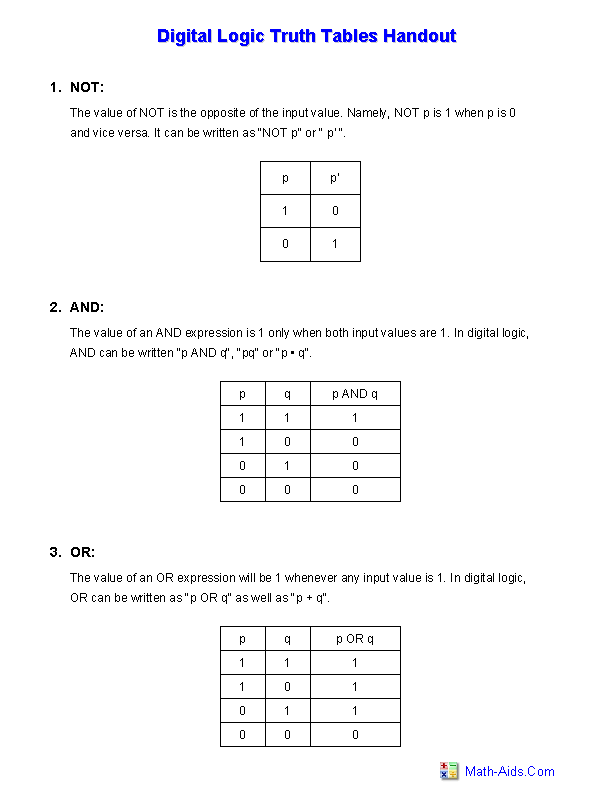Complete Truth TablesLogic Worksheets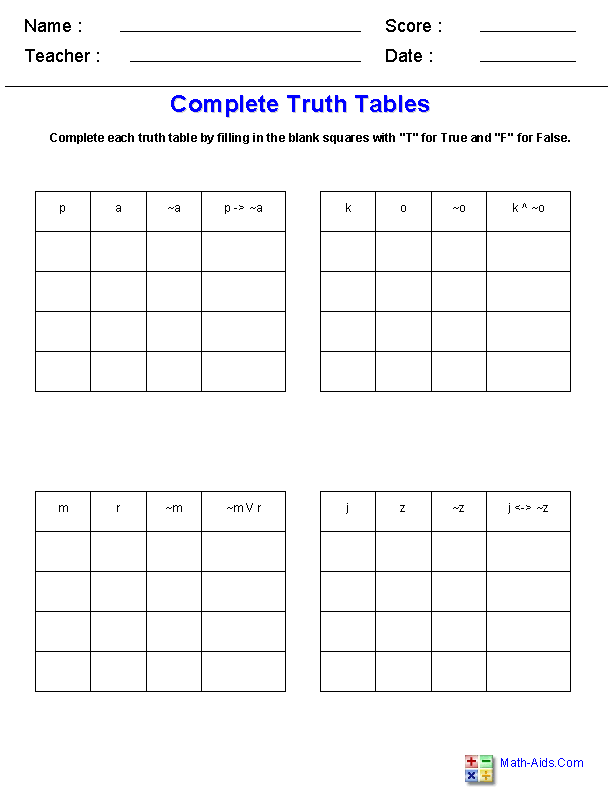Determine Truth ValuesLogic Worksheets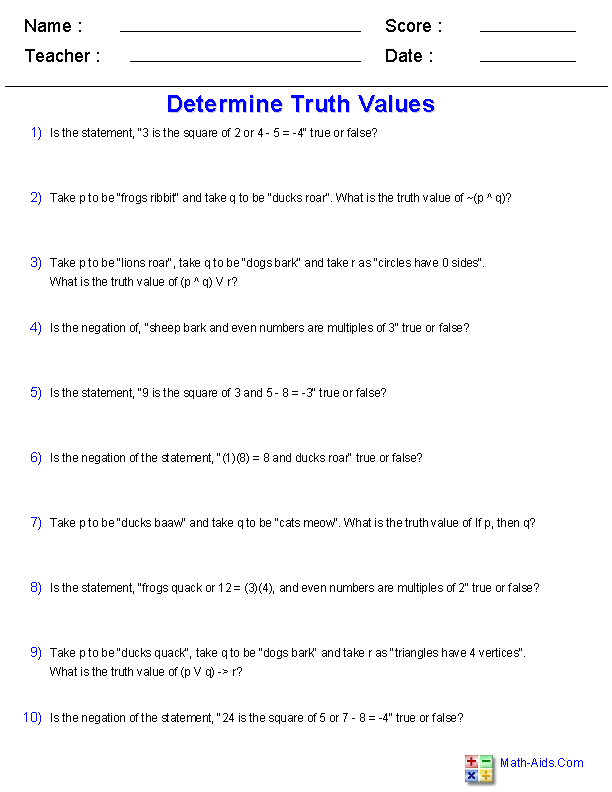Draw Truth TablesLogic WorksheetsLogic Truth Table ReviewLogic Worksheets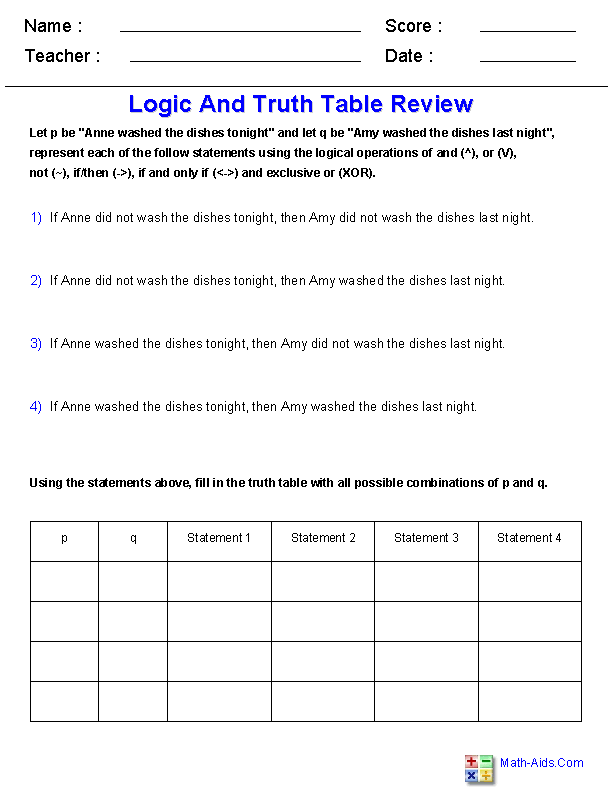Law of DetachmentLogic WorksheetsRecommended Videos

Detailed Description for All Logic Worksheets

Logic Definitions Handout
This Logic Definitions Handout will produce a handout that gives definitions and examples of important topics in logic. These Logic Worksheets are a good resource for students in the 8th Grade through the 12th Grade.

Truth Table Handout
This Logic Truth Table Handout will produce a handout that gives a brief explanation as well as truth tables of logical operations that are used when evaluating logic statements. These Logic Worksheets are a good resource for students in the 8th Grade through the 12th Grade.

Digital Truth Table Handout
This Logic Truth Table Handout will produce a handout that gives a brief explanation as well as truth tables of logical operations that are used predominately in digital logic. These Logic Worksheets are a good resource for students in the 8th Grade through the 12th Grade.

Complete Truth Tables Worksheets
These Logic Truth Table Worksheets will produce multiple truth tables of logic expressions that the student must fill in correctly. These Logic Worksheets are a good resource for students in the 8th Grade through the 12th Grade.

Determine Truth Values Worksheets
These Logic Truth Table Worksheets will produce multiple compound sentences that the student must determine the truth value of. These Logic Worksheets are a good resource for students in the 8th Grade through the 12th Grade.

Draw Truth Tables Worksheets
These Logic Truth Table Worksheets will produce multiple logic expressions that the student must draw and fill in correctly. These Logic Worksheets are a good resource for students in the 8th Grade through the 12th Grade.

Logic and Truth Table Review Worksheets
These Logic Truth Table Worksheets will produce a sheet in which the student must write out propositional expressions and fill in a truth table based on given statements. These Logic Worksheets are a good resource for students in the 8th Grade through the 12th Grade.

Law of Detachment Worksheets
These Logic Truth Table Worksheets will produce eight examples in which the student must use the law of detachment to draw a conclusion. These Logic Worksheets are a good resource for students in the 8th Grade through the 12th Grade.